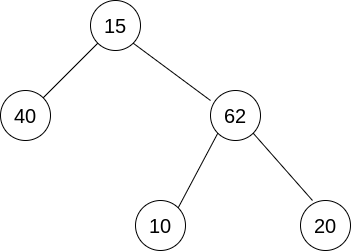New update is available. Click here to update.

# List of Depths

Posted: 9 Mar, 2021
Difficulty: Easy

## PROBLEM STATEMENT

#### You are given a binary tree. Your task is to convert this binary tree into a linked list, such that the value at nodes is in the level order form.

##### Input Format:
``````The first line of the input contains ‘T’ denoting the number of test cases.

The first line of each test case contains elements of the tree in the level order form. The line consists of values of nodes separated by a single space. In case a node is null, we take -1 in its place.
````````````For example, the level order input for the tree depicted in the above image would be :

15
40 62
-1 -1 10 20
-1 -1 -1 -1
``````

#### Explanation :

``````Level 1 :
The root node of the tree is 15.

Level 2 :
Left child of 15 = 40
Right child of 15 = 62

Level 3 :
Left child of 40 = null (-1)
Right child of 40 = null (-1)
Left child of 62 = 10
Right child of 62 = 20

Level 4 :
Left child of 10 = null (-1)
Right child of 10 = null (-1)
Left child of 20 = null (-1)
Right child of 20 = null (-1)
``````
##### Note :
``````The above format was just to provide clarity on how the input is formed for a given tree.

The sequence will be put together in a single line separated by a single space. Hence, for the above-depicted tree, the input will be given as:

15 40 62 -1 -1 10 20 -1 -1 -1 -1
``````
##### Output Format:
``````Just return the pointer to the head node of the linked list, no need to print anything,
``````
##### Constraints:
``````1 <= T <= 10
1 <= N <= 5 * 10^3
-1 <= A[i] <= 10^5

Time Limit: 1 sec
``````
SIMILAR PROBLEMS

Min Heap

Posted: 5 May, 2022
Difficulty: Moderate

Locked Binary Tree

Posted: 12 May, 2022
Difficulty: Easy

Left Right Print

Posted: 9 Jul, 2022
Difficulty: Moderate

The Summit

Posted: 15 Sep, 2022
Difficulty: Easy

Distance to a Cycle in Undirected Graph

Posted: 7 Nov, 2022
Difficulty: Moderate